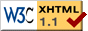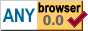Backreferences

# Backreferences

2021-05-25

## Introduction

One of the most commonly used techniques in data compression is to use backreferences to avoid repeating a sequence that has already occurred in the data. Instead of repeating the same sequence of bytes, a shorter sequence is emitted that tells the decoder to repeat the bytes it has previously seen. For example,

`Attention, attention!`

May be shortened to

`Attention, a{8, 11}!`

where {8, 11} denotes copy 8 characters, starting 11 characters before the last one. This type of compression is often referred to as sliding-window compression, dictionary compression, or Lempel-Ziv compression (after the authors of the 1977 paper titled A Universal Algorithm for Sequential Data Compression).

## Representation

Any method we use to encode backreferences needs a way for the decompressor to distinguish backreferences from bytes to be copied literally. A simple way to accomplish this is to start both backreferences and literal runs with a byte indicating the length of the sequence, and in that byte, always set the most significant bit to 1 for backreferences and to 0 for literal runs. If we use two bytes to encode the distance for a backreference, the compressed version of our text may look like this:

 12 Attention, a 136 11 0 1 !

In the above, we use 3 bytes for the backreference. This means we must save at least 4 bytes for a backreference to be profitable. This can mean avoiding starting a literal run with length 3 or greater, avoiding having to include the last 4 or more bytes of a literal run that has already started, or avoiding 5 or more bytes in the middle of a literal run (in that case, the cost of the backreference is the 3 bytes to encode the backreference, plus 1 byte to start a new literal run). The latter scenario is illustrated in the example above.

## Decoding

The decoder for data encoded this way is pretty simple:

``````pub fn decode<IO: IOTrait + RepeatOutput>(io: &mut IO) -> BoxResult<()> {
while let Some(b) = io.next_byte()? {
if b < 128 {
io.copy_bytes(b as usize)?;
} else {
let lo = io.next_byte()?.expect("end of input inside backreference");
let hi = io.next_byte()?.expect("end of input inside backreference");
let dist = ((hi as usize) << 8) | lo as usize;
io.repeat_bytes((b & 0x7f) as usize, dist)?;
}
}
Ok(())
}``````

Where `IOTrait` provides methods to read bytes from our input and write to our output, and `RepeatOutput` provides the `repeat_bytes` method that sends some previously-output bytes to the output again.

### Finding Repetitions

We can find repetitions in the data by maintaining a rolling hash of the last couple of bytes we have seen in the input. We then use that hash value in a lookup table which contains the most recent position at which we have seen that hash value. Here is a a simple implementation of that idea:

``````struct EncoderState {
// We find repititions by computing a rolling hash of the most recently
// seen 3 bytes.
hash: u32,

// We limit the magnitude of hash by bitwise anding with this mask.

// For each hash value, we record the most recent position at which
// we have encountered it.
pos: std::vec::Vec::<u64>,

// The literal length we have accumulated so far.
litlen: u8,
}

impl EncoderState {
/// Updates the hash value, records the position at which we encountered
/// it, and returns the previously most recent position for the same
/// hash value.
fn update_hash(&mut self, b: u8, pos: u64) -> u64 {
self.hash = ((self.hash << 5) ^ b as u32) & self.hash_mask;
let prev = self.pos[self.hash as usize];
self.pos[self.hash as usize] = pos;
prev
}

// ... impl EncoderState continues later``````

This `update_hash` function is used by a `find_rep` function, which finds the next repetition in the input (if any) and returns its length and distance, as well as the number of bytes before it that were not part of the repetition:

``````    // impl EncoderState {

fn find_rep<IO: IOTrait + LookbackInput>(&mut self, io: &mut IO)
-> BoxResult<(u8, u8, u64)> {
while let Some(b) = io.next_byte()? {
let pos = io.inpos();
let prev = self.update_hash(b, pos);
// Only return matches of length at least 3 that occur within
// 0x10000 bytes of the current position.
if prev >= 3 && (pos < 0x10000 || prev > pos - 0x10000) &&
io.lookback(prev - 3) == io.lookback(pos - 3) &&
io.lookback(prev - 2) == io.lookback(pos - 2) &&
io.lookback(prev - 1) == io.lookback(pos - 1)
{
return self.found_rep(io, pos, prev);
}
self.litlen += 1;
if self.litlen == 127 {
self.litlen = 0;
return Ok((127, 0, 0))
}
}

let litlen = self.litlen;
self.litlen = 0;
Ok((litlen, 0, 0))
}

// ... impl EncoderState continues later``````

This uses a helper function, `found_rep`, which counts the length of the repeated sequence and does some bookkeeping to keep the hash updated and ensure that a non-matching byte is correctly counted towards the next literal. Here it is:

``````    // impl EncoderState {

fn found_rep<IO: IOTrait + LookbackInput>(&mut self, io: &mut IO, pos: u64, prev: u64)
-> BoxResult<(u8, u8, u64)> {
let litlen_before = if self.litlen > 2 { self.litlen - 2 } else { 0 };
let dist = pos - prev - 1;
let mut matlen = 3;
let mut prevpos = prev;
self.litlen = 0;
while let Some(b) = io.next_byte()? {
self.update_hash(b, io.inpos() - 1);
if b == io.lookback(prevpos) {
matlen += 1;
prevpos += 1;
} else {
self.litlen = 1;
break;
}
if matlen == 127 { break };
}
return Ok((litlen_before, matlen, dist));
}

// ... impl EncoderState continues later``````

Finally, we have a `new` function for convenience:

``````    // impl EncoderState {

fn new() -> EncoderState {
let mask = (1 << 14) - 1;
let mut pos_table = Vec::new();
EncoderState {
hash: 0,
pos: pos_table,
litlen: 0,
}
}
}``````

The encoder creates an `EncoderState` and uses it to repeatedly find literals and matches until all input has been consumed. The matches and literals are encoded as specified by the file format:

``````pub fn encode<IO: IOTrait + LookbackInput>(io: &mut IO) -> BoxResult<()> {
let mut state = EncoderState::new();
loop {
let pos = io.inpos() - state.litlen as u64;
let (litlen, matlen, dist) = state.find_rep(io)?;
if litlen == 0 && matlen == 0 { return Ok(()) }
if litlen > 0 {
write_lit(io, litlen, pos)?;
}
if matlen > 0 {
io.write_byte(0x80 + matlen)?;
io.write_byte((dist & 0xff) as u8)?;
io.write_byte((dist >> 8) as u8)?;
}
}
}

fn write_lit<IO: IOTrait + LookbackInput>(io: &mut IO, litlen: u8, start: u64) -> BoxResult<()> {
io.write_byte(litlen)?;
let mut pos = start;
for _ in 0..litlen {
io.write_byte(io.lookback(pos))?;
pos += 1;
}
Ok(())
}``````

## Improvements

Both the representation of compressed data and the algorithm for producing compressed data have been designed primarily to facilitate illustration. Better compression can be achieved with some modifications. For example:

• As written, the encoder only maintains a single last seen position for each hash value. It is possible that a longer repetition exists at an earlier position. To make use of this, the encoder could be adapted to remember more than just the most recent position at which a hash value occurred.
• An interesting alternative is to only remember a single occurrence of each hash value (as the encoder described here does), and take advantage of this property by omitting the distance of the match. The decoder can reconstruct the position of the match by performing the same hash calculations that the encoder does. This is called LZP compression (Lempel-Ziv Predictive) and was first described by Charles Bloom.
• Yet another alternative is to both remember multiple positions where a hash value occurred and do the same computation in the decoder, and change the encoding to specify which of these positions to use using a few bits, instead of the 16 bit the example encoding uses.
• Instead of starting with no data to look back on, we could logically precede the data with some commonly encountered byte sequences. For example, if we are compressing English text, we could include some common words (that are long enough to warrant a backreference). If we are compressing HTML, we could include some HTML tags. If we don't know in advance what we are compressing, we could include a mixture of things.
• In data encountered in practice, lengths are often small, and repetitions are common. This means we can do better than the "one bit to distinguish literals and repetitions, 7 bits to encode length" encoding used here. The observation that some values are more common than others also forms a neat bridge to another big topic in data compression: entropy coding.

## Example Code

A complete decoder and encoder using the example code here is provided in the example code project.